Note: The other languages of the website are Google-translated. Back to English

## How to sum multiple columns based on single criteria in Excel?

In Excel, you may always need to sum multiple columns based on one criteria. For example, I have a range of data as following screenshot shown, now, I want to get the total values of KTE in three months - Jan, Feb and Mar.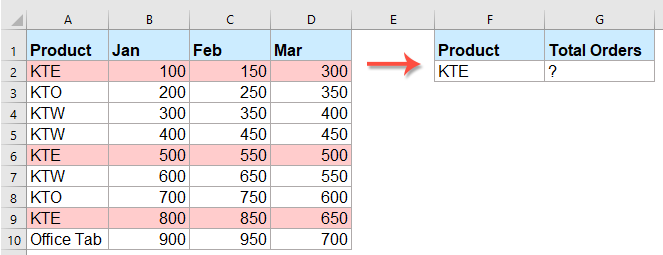Sum multiple columns based on single criteria with a helper column

Sum multiple columns based on single criteria with an array formula

Sum multiple columns based on single criteria with an awesome feature

#### Sum multiple columns based on single criteria with a helper column

In Excel, we can create a helper column to sum the total values for each row, and then use the sumif function to sum this column based on criteria, please do as this:

1. In this example, you can sum the total values for each row first, please type this formula: =sum(B2:D2), then drag the fill handle down to the cells that you want to apply this formula, and the total values of each row will be displayedsee screenshot: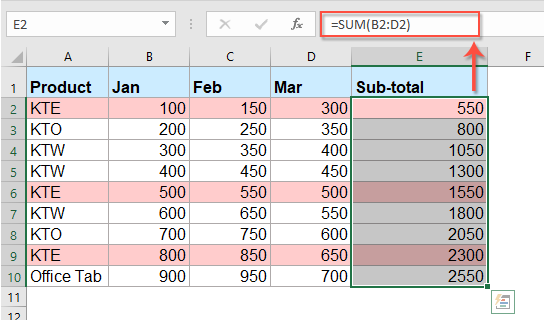2. And next, you can apply the below sumif function to sum the data in helper column E based on the criteria:

=SUMIF(A2:A10, G2, E2:E10)

Then, press Enter key on the keyboard, and you will get the total number based on the specific criteria. See screenshot: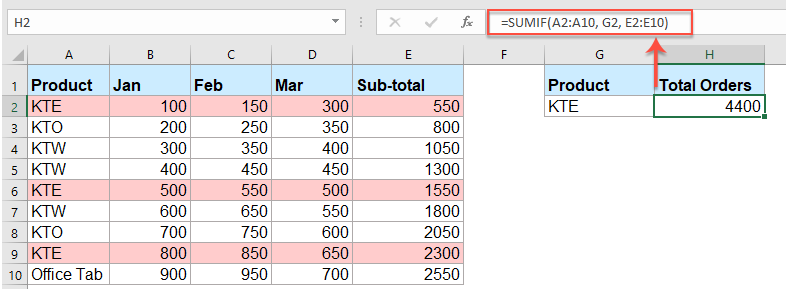Tips: In the above formula：

• A2:A10 refers to the range of cells that you want to apply the criteria against;
• G2 is the criterion that the items are to be added;
• E2:E10 specifies the cells to be added.

lookup and sum all the matching values in rows or columns

Kutools for Excel's LOOKUP and Sum feature helps you to lookup the specific value and get the summation of all the matching values in rows or columns as you need. Click to download Kutools for Excel!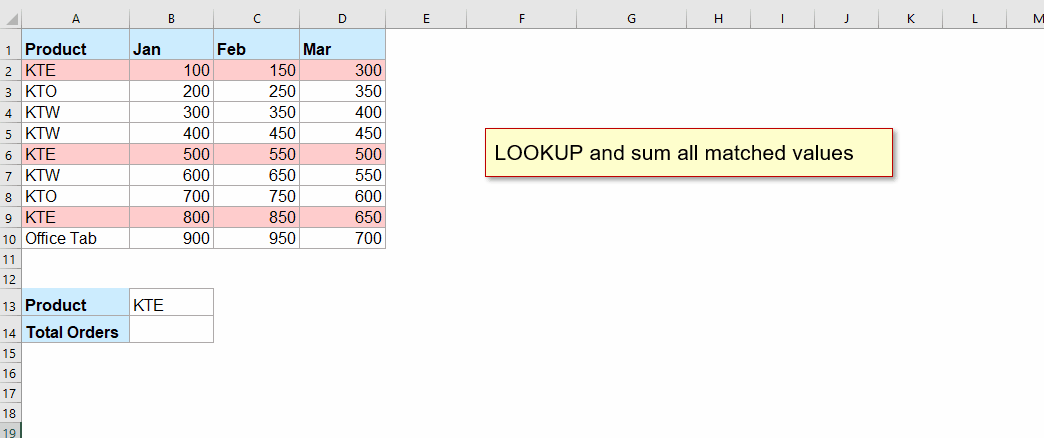Kutools for Excel: with more than 300 handy Excel add-ins, free to try with no limitation in 30 days. Download and free trial Now!

#### Sum multiple columns based on single criteria with an array formula

If you don’t like to use the helper column to solve this problem, here also is an array formula that can do you a favor.

1. Enter or copy the following formula in your specific cell - G2:

=SUM((B2:B10+C2:C10+D2:D10)*(--(A2:A10=F2)))

2. And then press the Ctrl + Shift +Enter keys together, and you will get the correct result.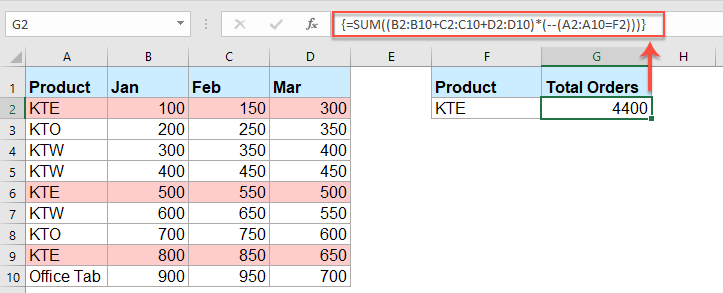Tips: In the above formula：

• B2:B10, C2:C10 and D2:D10, indicate the columns that you want to sum, if you have more columns data need to sum, just add the column range as your need;
• A2:A10 refers to the range of cells that you want to apply the criteria against;
• F2 is the criterion that the items are to be added.

#### Sum multiple columns based on single criteria with an awesome feature

to deal with this task as quickly as possible, Kutools for Excel's LOOKUP and Sum feature also can help you.

Tips:To apply this LOOKUP from Bottom to Top feature, firstly, you should download the Kutools for Excel, and then apply the feature quickly and easily.

After installing Kutools for Excel, please do as this:

1. Click Kutools > Super LOOKUP > LOOKUP and Sum, see screenshot: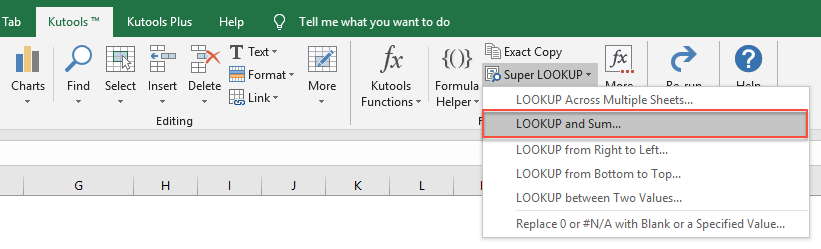2. In the LOOKUP and Sum dialog box, please do the following operations:

• Select Lookup and sum matched value(s) in row(s) option under the Lookup and Sum Type section;
• Specify the lookup value, output range and the data range that you want to use;
• Select Return the sum of all matched values option from the Options.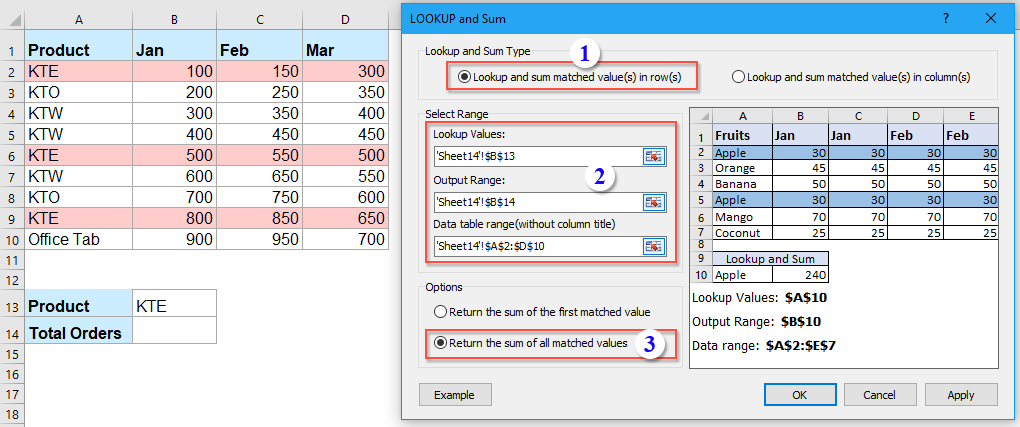3. Then, click OK button, and the total value of all the matched records has been calculated at once, see screenshot: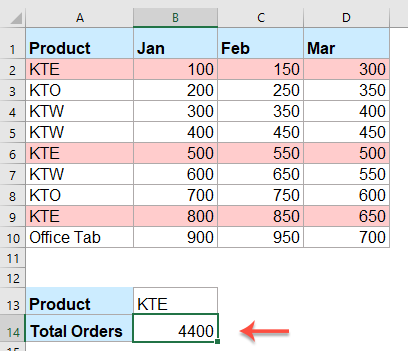#### More relative articles:

• Sumif with multiple criteria in one column
• In Excel, SUMIF function is a useful function for us to sum cells with multiple criteria in different columns, but with this function, we can also sum cells based on multiple criteria in one column. In this article. I will talk about how to sum values with more than one criteria in same column.
• Sumif with one or more criteria in Excel
• In Excel, sum values based on one or more criteria is a common task for most of us, the SUMIF function can help us to quickly sum the values based on one condition and the SUMIFS function help us to sum values with multiple criteria. This article, I will describe how to sum with one or more criteria in Excel?
• Sumif Cell Values Between Two Given Dates In Google Sheets
• In my Google sheet, I have two columns which contain a date column and order column, now, I want to sum the order column cells based on the date column. For example, sum values between 2018/5/15 and 2018/5/22 as following screenshot shown. How could you solve this job in Google sheets?
• Sum Cells When Value Changes In Another Column
• When you work on Excel worksheet, sometime, you may need to sum cells based on group of data in another column. For example, here, I want to sum the orders in column B when the data changes in column A to get the following result. How could you solve this problem in Excel?
• Vlookup Across Multiple Sheets And Sum Results In Excel
• Supposing, I have four worksheets which have the same formatting, and now, I want to find the TV set in the Product column of each sheet, and get the total number of order across those sheets as following screenshot shown. How could I solve this problem with an easy and quick method in Excel?

### The Best Office Productivity Tools

#### Kutools for Excel Solves Most of Your Problems, and Increases Your Productivity by 80%

• Reuse: Quickly insert complex formulas, charts and anything that you have used before; Encrypt Cells with password; Create Mailing List and send emails...
• Super Formula Bar (easily edit multiple lines of text and formula); Reading Layout (easily read and edit large numbers of cells); Paste to Filtered Range...
• Merge Cells/Rows/Columns without losing Data; Split Cells Content; Combine Duplicate Rows/Columns... Prevent Duplicate Cells; Compare Ranges...
• Select Duplicate or Unique Rows; Select Blank Rows (all cells are empty); Super Find and Fuzzy Find in Many Workbooks; Random Select...
• Exact Copy Multiple Cells without changing formula reference; Auto Create References to Multiple Sheets; Insert Bullets, Check Boxes and more...
• Extract Text, Add Text, Remove by Position, Remove Space; Create and Print Paging Subtotals; Convert Between Cells Content and Comments...
• Super Filter (save and apply filter schemes to other sheets); Advanced Sort by month/week/day, frequency and more; Special Filter by bold, italic...
• Combine Workbooks and WorkSheets; Merge Tables based on key columns; Split Data into Multiple Sheets; Batch Convert xls, xlsx and PDF...
• More than 300 powerful features. Supports Office/Excel 2007-2019 and 365. Supports all languages. Easy deploying in your enterprise or organization. Full features 30-day free trial. 60-day money back guarantee.#### Office Tab Brings Tabbed interface to Office, and Make Your Work Much Easier

• Enable tabbed editing and reading in Word, Excel, PowerPoint, Publisher, Access, Visio and Project.
• Open and create multiple documents in new tabs of the same window, rather than in new windows.
• Increases your productivity by 50%, and reduces hundreds of mouse clicks for you every day!No ratings yet. Be the first to rate!
This comment was minimized by the moderator on the site
How do you sum with multiple criteria in single column and sum range in multiple columns
This comment was minimized by the moderator on the site
Try the formula Sumifs
This comment was minimized by the moderator on the site
How can I sum multiple columns from single criteria/range? This formula doesn't work but will hopefully explain what I want. =SUMIF(C3:C36,C40,D3:W36) The result of this formula only SUMs D3:D36 and not the extra colums, i.e. E3:E36
This comment was minimized by the moderator on the site
Can you help me get rid of the Div/0 in this formula. #DIV/0. =IF((F9+G9)>E65,E65/(F9+G9)*2,E65/(F9+G9+H9)*3)
This comment was minimized by the moderator on the site
This comment was minimized by the moderator on the site
How can I sum multiple columns from single criteria/range?Can anyone advise using the below formula

=sumif(T12:T69,>0,(sum(G13:G69)*sum(T12:T69)))
This comment was minimized by the moderator on the site
Sum all column values that has a specific value in the first column

Cell A1: a
Cell B1: {=SUM(--(A1=A3:A9)*B3:F9)} resulting in the sum 104 (has to be entered by using CTRL+SHIFT+ENTER)

a 104

a 2 5 7 2 2
b 5 1 6 9 4
c 7 6 1 1 9
a 4 5 8 6 2
b 4 8 3 2 6
a 6 9 4 8 5
a 6 8 9 5 1
This comment was minimized by the moderator on the site
Hi

I have used the formula outlined in "Sum multiple columns based on single criteria with an array formula" - I am working with tables and address the columns with the table column names - the formula works however only if the criteria is YES for the first row in the table - if the criteria is not matched the total Sum is equal to Zero - it seems to disregard the other rows and does no sum them up. - any idea?

Nicole
This comment was minimized by the moderator on the site
Gracias por su publicacion! Me ayudo mucho!!!
esra tan
simple! jeje Gracias!
This comment was minimized by the moderator on the site
This worked for me however I don't understand what the (-- is for?
This comment was minimized by the moderator on the site
i need 1+1+1+1 in one column total in auto
There are no comments posted here yet